Tuesday 29th September 2020

CBSE Guess > Papers > Question Papers > Class XII > 2003 > Chemistry > Outside Delhi Set -I

CHEMISTRY—2003 (Set I—Outside Delhi)

Q. 1. Name one ion whose central atom has thesp type of hybrid orbitals. 1

Q. 2. Name a salt that can be added to AgCI so as to produce cation vacancies. 1

Q. 3. Which radioactive series starts from U-235 and terminates at Pb-207? 1

Q. 4. What is meant by 'shape selective' catalysis? 1

Q. 5. Why is the bond dissociation energy of fluorine molecule less than that of chlorine molecule? 1

Q. 6. What is the effect of increasing pH on K Cr 2O 7 solution? 1

Q. 7. Name the following complex using IUPAC norms:1

Q. 8. Why is cellulose in our diet not nourishing? 1

Q. 9. Give one example each of (a) a vat dye, (b) a mordant dye. 1

Q. 10. Mention the composition of a composite propellant. 1

Q. 11. Br - ions form close packed structure. If the radius of Br - ion is 195 pm, calculate the radius of the cation that just fits in the tetrahedral hole.
Can a cation A + having a radius of 82 pm be slipped into the Octahedral hole of the crystal2

Q. 12. Carbon tetrachloride and water are immiscible whereas ethanol and water are miscible in all proportions. Correlate this behaviour with molecular structures of these compounds. 2

Q. 13. What is meant by bond order? Calculate the bond orders of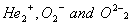molecular Ions. 2

Q. 14. Why does the molar conductance increase on diluting the solution of a weak electrolyte? Electrolytic conductivity of 0.30 M solution of KCI at 298 K is 3.72 x 10 -2 S cm -1 . Calculate its molar conductivity. 2

Q. 15. (a) State the factors that influence the value of cell potential of the following cell: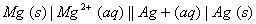(b) Write Nernst equation to calculate the cell potential of the above cell. 2

Q. 16. What is known as 'activation energy'? How is the activation energy affected by (i) the use of a catalyst and (ii) a rise in temperature? 2

Q. 17. The reaction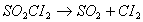is a first order reaction with half-life 3.15 x 10 4 s at 320 0 C. What percentage of SO2 Cl 2 would be decomposed on heating at 3200C for 90 minutes? 2

Q. 18. Calculate the energy released (in joules) in the fusion reaction per atom of helium formed: 2Given: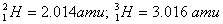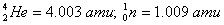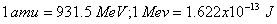Q. 19. What is adsorption? How does adsorption of a gas on a solid surface vary with (a) temperature and (b) pressure?
Illustrate With the help of appropriate graphs. 2

Q. 20. Write IUI'AC names of the following: 2

(i)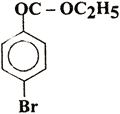(ii) CH 3 - C = C - CH 2 - CHO

Q. 21. Write one chemical equation each to exemplify the following reactions: 2
(i) Carbylamine reaction
(ii) Hofmann bromamide reaction

Q. 22. What are borones? How is diborane prepared on an industrial scale? Draw the structure of diborane molecule. 2

Q. 23. Draw the structure of ferrocene and write the reaction involved hi the preparation of ferrocene. 2

Q. 24. Write equations used for the synthesis of (i) terylene, (ii) neoprene. 2

Q. 25 . What are phospholipids? Give their important uses. 2

Q. 26. Name the components of blood which are responsible for: 2
(i) Blood clotting
(ii) Source of energy
(iii) Maintaining pH of blood within a suitable range
(iv) Defence against infection.

Q. 27. What is meant by the statement that 'an electron has dual nature'? Calculate the wavelength associated with a moving electron having a kinetic energy of 1.1375 x 10 -25 J. 3
[me = 9.1 x 10-31 kg: h = 6.6 x 10 -34 Js]

Q. 28. An aqueous solution containing 1.248 g of barium chloride (molar mass = 208.34 mol -1 ) in 100 g of water boils at 100.08320;C. Calculate the degree of dissociation of barium chloride.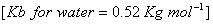3

Q. 29. How is a change in free energy related to the spontaneity of a reaction? Calculate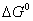of the following reaction? 3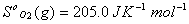Q. 30. Complete the following reactions: 3Q. 31. How is aniline prepared on a large scale? How will you convert it into: 3
(i) Benzonitrile,
(ii) Acetanilide?
Write the reaction and the conditions in each case.

Q. 32. Account for the following: 3
(a) Tendency to show -2 oxidation state diminishes from sulphur to polonium in Group 16.
(b) Boron forms electron deficient compounds.
(c) PbCI4 is less stable than SnCl 4 .

Q. 33. (a) Describe the preparation of acetic acid from acetlyene.
(b) How can the following be obtained from acetic acid:
(i) Acetone
(ii) Acetaldehyde
(c) In what way can acetic acid be distinguished from acetone?
(d) Why do carboxylic acids not give the characteristic reactions of a carbonyl group? 5

Q. 34. (a) What is the basic difference between the electronic configurations of the transition and inner transition elements?
(b) Discuss the general trends in the following properties of the 3d transition elements (21 - 29):
(i) Atomic size
(ii) Oxidation states
(iii) Formation of coloured ions

 Chemistry 2003 Question Papers Class XII Delhi Outside Delhi Compartment Delhi Compartment Outside DelhiSet ISet ISet ISet ISet IISet IISet IISet II

CBSE 2003 Question Papers Class XII